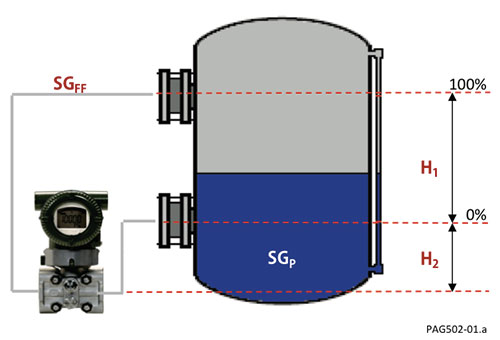Bernoulli’s equation states that the pressure drop across a constriction is proportional to the square of the flow rate. Using this equation, smart differential pressure transmitters can infer the flow.

In this type of application, it is not only important to have an accurate differential pressure transmitter, but also an accurate “constriction”. It is the accuracy of the total system that must be considered.

Therefore, Yokogawa combines our DP transmitter with an Integral Flow Orifice (IFO) in one unit to produce a total system to measure low flow accurately.Differential Pressure Transmitter attached to an IFO assembly based on the EJA-E Series.Differential Pressure Transmitter attached to an IFO assembly based on the EJX-A Series.

### Which Series is Right for You?

EJA115E EJX115A Accuracy• • • • • • • • • • • • • •

### Introduction

Level transmitter configuration can be very time consuming. Calculations required to determine proper range values for traditional transmitters can become complex due to the physical layout of an application.

With maintenance shops getting smaller, finding equipment that allows us to do more with fens becomes a priority. DPharp transmitters with advanced software functionality can eliminate these complex calculations.

### Application

Using typical smart or conventional products, all the following must be considered:

 The specific gravity of the process SGP Precise location of 0% and 100%Specific Gravity of the capillary fill fluid (or sealing fluid used in impulse piping) SGFF Exact orientation oi the transmitter to the vessel H2 Vertical distance between the process conn. H1

Depending on the application, the vessel may be open (referencing atmosphere) or closed (under a blanket pressure).

Elevation is typically used when the vessel is closed. To reference the blanket pressure, a low side remote seal may be used (or a wet leg). The capillary on remote seal creates a negative force on the transmitter equal to the vertical height times the specific gravity of the fill fluid.

Elevation = (H1 + H2) x SGFF

Suppression is a positive pressure created on the high-pressure side of the transmitter typically due to the transmitter being positioned below the 0% process connection. Suppression is present in both open arid closed vessels. Suppression is equal to the vertical distance between the 0% process connection and the transmitter times the Specific Gravity of the fill livid.

Suppression = H2 x SGFF

Span is the vertical distance between the process connections times the process medium’s Specific Gravity.

Span = H1 x SGPFigure 1: Closed Tank

Now that you have the ElevationSuppression, and Span, the calibration values can be calculated for the 0% (Empty) arid the 100% (Full).

Cal Value (0%) = Suppression – Elevation

Cal Value (100%) = (Suppression + Span) – Elevation

Example: (using figure 1)

 SGP 0.9 H2 10 inches SGFF 0.8 H1 20 inches

Cal Value 0%) = Suppression – Elevation
Cal Value (0%) = (H2 x SGFF) – (H1 + H2 x SGFF)
Cal Value (0%) = (10 x 0.8) – (20 + 10) x 0.8
Cal Value (0%) = 8 – 24
Cal Value 0%) = -16 inH2O

Cal Value (100%) = (Suppression + Span) – Elevation
Cal Value (100%) = ((H2 x SGFF) + (H1 x SGP)) – (H1 + H2) x SGFF
Cal Value (100%) = ((10 x 0.8) + (20 x 0.9)) – (20 + 10) x 0.8
Cal Value (100%) = (8 + 18) – 24
Cal Value (100%) = +2 inH2O

Therefore, Calibrated Range would be:

 -16 inH2O +2 inH2O 0% 100% Empty Full